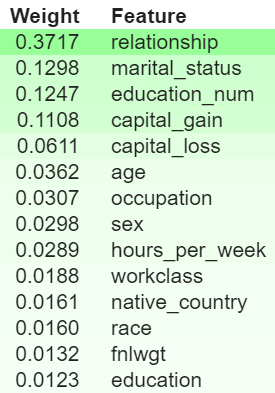# Feature Importance with OneHotEncoder and Pipelines in Scikit-learnI started using pipelines to stream-line machine learning model training process, and now I quite like using Pipelines. It tooks more a couple of hours, however, to discover how to see the most important features for the models trained when I have used a pipeline. Let me first explain how the pipelines work, and then I will show how to easily see the most important features.

### Training Pipelines

1. First I want to understand what type of variables are held in each dataframe column - numeric, continuous, categorical or boolean. Here I am exploring a house price dataset with variables house price, location, age, interest, interest rate, year. For a description of the problem I have chosen - read here.
``````X_train # training dataframe

# a. check the types of the columns
[In]: X_train.dtype

[Out]:
house price      float64
location         category
age              int64
interest         float64
interest rate    object
year             period[M]

``````
1. After I know this I create two arrays in which I hold the names of the dataframe columns with numeric and categorical values.
``````# b. get the names of the numeric anc categorical columns
numeric_features = X_train.select_dtypes(include=['int64', 'float64']).columns
categorical_features = X_train.select_dtypes(include=['object', 'category', 'period[M]']).columns
``````

Here is how my training pipelines looks like:

1. I to encore the categorical valiables - and I choose OneHotEncoder to do this.

Let’s imagine a very simplistic scenario. Let’s say that I have data only for houses purchased in 2017, 2018 and 2019. I have chosen to treat the variable year as a datetime type instead of numerical. Let’s also assume that I have data for only 5 houses. The values of the variable year would look something like this:

``````[In]: X_train.year

[Out]:
index    year
0        2017
1        2019
2        2019
3        2018
4        2017
``````

OneHotEncoder transforms the column year into another 3 binary (and numeric for False is 0, and True is 1) columns names year_2017, year_2018 and year_2019. Each of this columns will have the value of 1 when the house is bought during the year in its title, and 0 otherwise. The column year would then not be used during training (but is listed below for simplicity).

``````[In]: X_train.year

[Out]:
index    year    year_2017    year_2018    year_2019
0        2017            1            0            0
1        2019            0            0            1
2        2019            0            0            1
3        2018            0            1            0
4        2017            1            0            0
``````

Let’s finally apply OneHotEncoder to the data set, while imputing (or filling) missing values. Because I will do 2 things here already - encoding and imputing, I will do these using a pipeline. Note: Scikit-learn does not allow training with datasets, which have missing values - and if they do, an error is thrown during training.

``````# import the needed libraries first
from sklearn.pipeline import Pipeline
from sklearn.impute import SimpleImputer
from sklearn.preprocessing import StandardScaler, OneHotEncoder

# create a transformer for the categorical values
categorical_transformer = Pipeline(steps=[
('imputer', SimpleImputer(strategy='constant', fill_value='missing')),
('one_hot', OneHotEncoder())])

# create a transformed for the numerical values
numeric_transformer = Pipeline(steps=[
('imputer', SimpleImputer(strategy='median')),
('scaler', StandardScaler())])
``````

Note: The numerical columns are also encored here - they are scaled and all missing values replaced wit the median value for each variable.

Finally, apply these transformations to call columns (variables) in the dataset

``````from sklearn.compose import ColumnTransformer

preprocessor = ColumnTransformer(
transformers=[
('num', numeric_transformer, numeric_features),
('cat', categorical_transformer, categorical_features)
])
``````
1. Train a Linear Regression (or a model of your choise) using a pipeline with a preprocessing step, and a classification step.
``````clf = Pipeline(steps=[('preprocessor', preprocessor),
('classifier', LinearRegression())])
``````

Perfect, I now have a trained Linear Regression model, which I’d like to see feature important for.

### Feature Importance in Pipelines: Problem

Usually, I would get the `coef_` of a scikit-learn trained model, which will give me the weights for each of the features (variables or columns). The problem here is that OneHotEncoder creates new features, as shown above when discussing OneHotEncoder. I am not aware of what the names of these features are and which of the weights of `coef_` corresponds to each of the new features.

This cannot be right as a data scientist I must know which features and important for the model.

### Feature Importance in Pipelines: Solution

1. Get OneHotEncoder column names using `named_steps` of the training pipeline

As you see above, I have named the OneHotEncoding step as one_hot, the Linear Regression training step and classifier. `named_steps` allows me to get only one of all pipeline steps and inspect it alone. What I want to do first is to get the names of the columns which were created during OneHotEncoding.

To do this, I need to get to the preprocessor step, call the categorical transformer cat, and get the OneHotEncoder step one_hot

``````[In]: onehot_columns = list(clf.named_steps['preprocessor'].named_transformers_['cat'].named_steps['one_hot'].get_feature_names(input_features=categorical_features))

[Out]:
['year_2017', 'year_2018', 'year_2019', 'location_USA', ...., 'location_Brazil']
``````
1. Join the lists of the numerical column names and the OneHotEncoder column names
``````numeric_features_list = list(numeric_features)
numeric_features_list.extend(onehot_columns)
``````
1. Use the magic library ELI5 (or ‘explain like I’m 5’)
``````eli5.explain_weights(clf.named_steps['classifier'], top=50, feature_names=numeric_features_list, feature_filter=lambda x: x != '<BIAS>')
``````

The output is a very beautiful list with the weight + column name is printed to show feature importance - all positive weights are in green, while the negative weights are visualized in red.

Here is a sample output of `eli5.explain_weights`:### Ralted Posts:

This post is a follow up to the Regression Tips anc Tricks Series I have made.